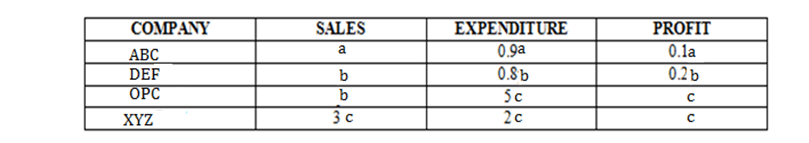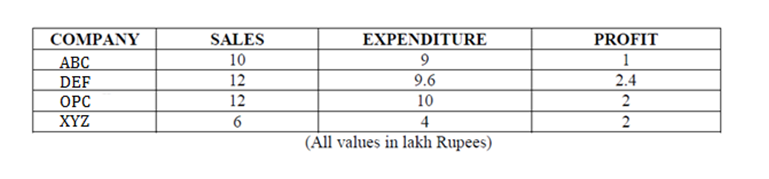DIRECTION : Ghosh Babu has recently acquired four companies namely (ABC), (DEF), (OPC) and (XYZ). When the results of the companies for the year 1992 – 93 were placed before him. He found a few interesting things about them. While the profits of OPC and XYZ were the same, the sales of OPC were the same as those of DEF . Profits of ABC were 10% of its sales, where as the profits of DEF were 20% of its sales. While the total expenses of OPC were 5 times its profits, sales of XYZ were 3 times its profits. The total expenses of OPC were Rs. 10,00,000, the total expenses of ABC were 10% less than those of OPC. Profits are defined as the difference between sales and total expenses.

Question 1. Which company had the highest total expenses?
(a) ABC
(b) DEF
(c) OPC
(d) XYZ

Question 2. Which company had the lowest sales?
(a) ABC
(b) DEF
(c) OPC
(d) XYZ

Question 3. Which company had the highest profits?
(a) ABC
(b) DEF
(c) OPC
(d) XYZ

Question 4. Which company had the lowest profits?
(a) ABC
(b) DEF
(c) OPC
(d) XYZ

Let the profits of OPC & XYZ be c, Sales of OPC & DEF = b and sales of ABC = a. So we haveNo, it is said that the total expenses of OPC were Rs.10 lakhs. Hence 5c = Rs.10 lakhs or c = Rs.2 lakhs. Also Total expenses of ABC were 10% less than those of OPC = Rs.9 lakhs. Hence 0.9a = 9 lakhs or a = 10 lakhs. Finally, In case of OPC, since Sales – Expenditure = Profit, Sales = Expenditure + Profit = 6b = 12 lakhs. Hence b = 12 lakhs. So our table is modified to :OPC had highest total expenses i.e. Rs. 10 lakhs.

From the above table it can be seen that the company that had the lowest sales is XYZ viz. Rs.6 lakhs.